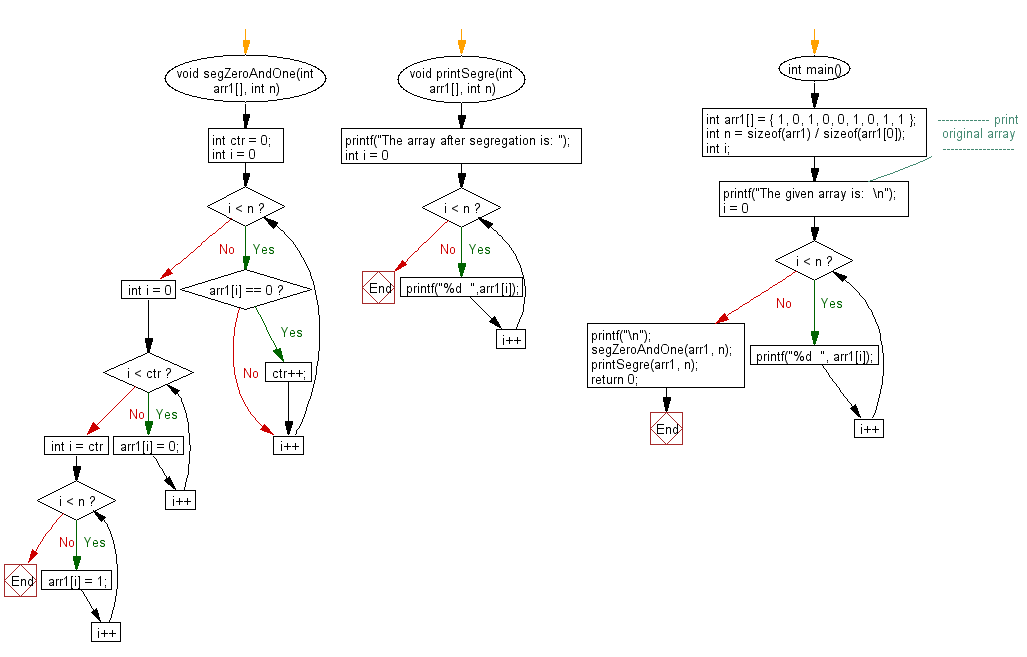﻿ C exercises: Segregate 0s and 1s in an array - w3resource# C Exercises: Segregate 0s and 1s in an array

## C Array: Exercise-95 with Solution

Write a program in C to segregate 0s and 1s in an array.

Sample Solution:

C Code:

``````#include <stdio.h>

void segZeroAndOne(int arr1[], int n)
{
int ctr = 0;

for (int i = 0; i < n; i++) {
if (arr1[i] == 0)
ctr++;
}
for (int i = 0; i < ctr; i++)
arr1[i] = 0;

for (int i = ctr; i < n; i++)
arr1[i] = 1;
}
void printSegre(int arr1[], int n)
{
printf("The array after segregation is: ");
for (int i = 0; i < n; i++)
printf("%d  ",arr1[i]);
}
int main()
{
int arr1[] = { 1, 0, 1, 0, 0, 1, 0, 1, 1 };
int n = sizeof(arr1) / sizeof(arr1);
int i;
//------------- print original array ------------------
printf("The given array is:  \n");
for(i = 0; i < n; i++)
{
printf("%d  ", arr1[i]);
}
printf("\n");
//-----------------------------------------------------------
segZeroAndOne(arr1, n);
printSegre(arr1, n);

return 0;
}
```
```

Sample Output:

```The given array is:
1  0  1  0  0  1  0  1  1
The array after segregation is: 0  0  0  0  1  1  1  1  1
```

Pictorial Presentation:Flowchart:C Programming Code Editor:

Improve this sample solution and post your code through Disqus.

﻿